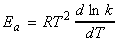Back to Table of Contents [TOP] Chemical Kinetics (ii)  The temperature dependence of reaction rates (Arrhenius equation) [ TOP ] (6-3) The temperature dependence of reaction rates (Arrhenius equation) The rate constants of most reactions increase as the temperature is raised, this increase being usually within the range spanned by: Lower end: the hydrolysis of methyl ethanoate. In this case we have: k(35oC) = 1.82 k(35oC)) Upper end: the hydrolysis of sucrose. In this case we have: k(35oC) = 4.13 k(35oC) In fact, as a rule of thumb, we usually assume that a 10oc increase in temperature will result in doubling of the rate of a reaction. The relationship between the rate constant k and temperature is an empirical law, known as the Arrhenius equation:or:where A is called the pre-exponential factor or the frequency factor, and Ea is the activation energy. Collectively, these two terms are known as the Arrhenius parameters and may be quoted from data books.  The 'log' form of the equation is particularly useful since a plot of ln k vs. 1/T is expected to be a straight line, with gradient -Ea / R and y-intercept or ln A. Note: The fact that Ea is given by the slope of the plot of ln kvs. 1/T signifies that the higher the activation energy, the stronger is the temperature dependence of the rate constant.  If the reaction has a zero activation energy, then the rate is independent of temperature. If the activation energy is negative, then the rate decreases with an increase of temperature. (This is possible, but it is an indication that the reaction mechanism is quite complex.) Note that the temperature dependence of some reactions is not Arrhenius-like, in the sense that a straight line is not obtained when ln k is plotted against 1/T. However, it is still possible to define an activation energy as:This new equation permits the treatment of systems with highly temperature-dependent activation energy. It is a more general form of the Arrhenius equation as it allows Ea to be obtained from the slope (at the temperature of interest) of a plot of ln k against 1/T even if the Arrhenius plot is not a straight line. Non-Arrhenius behaviour is commonly a sign that quantum mechanical tunnelling is playing a significant role in the reaction. NOTE:  Although until now we have regarded the Arrhenius parameters as purely empirical quantities, it is worth anticipating that the activation energy is the minimum kinetic energy that reactants must have in order to form products. For example, in a gas-phase reaction there are numerous collisions each second, but only a tiny proportion are sufficiently energetic to lead to reaction. The fraction of collisions with a kinetic energy in excess of an energy Ea is given by the Boltzmann distribution as e-Ea/RT. Hence, the exponential factor in the Arrhenius equation can be interpreted as the fraction of collisions that have enough kinetic energy to lead to reaction. The pre-exponential factor is a measure of the rate at which collisions occur irrespective of their energy. Hence, the product of A and the exponential factor, e-Ea/RT, gives the rate of successful collisions. An analogous argument can also be made for reactions in the liquid phase. All this is discussed at a later stage.